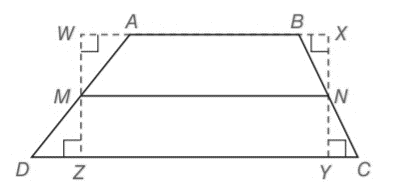Chapter 4.4, Problem 6E### Elementary Geometry for College St...

6th Edition
Daniel C. Alexander + 1 other
ISBN: 9781285195698

#### Solutions

Chapter
Section### Elementary Geometry for College St...

6th Edition
Daniel C. Alexander + 1 other
ISBN: 9781285195698
Textbook Problem
58 views

# In trapezoid ABCD, M N ¯ is the median. Without writing a formal proof explain why M N = 1 2 ( A B + D C ) .To determine

To explain:

Why MN=12(AB+DC).

Explanation

Consider the following trapezoid ABCD.

In a trapezoid ABCD, MN is the median.

We know that, the length of the median (m) of a trapezoid is the average of the lengths of the bases.

It means m=b

### Still sussing out bartleby?

Check out a sample textbook solution.

See a sample solution

#### The Solution to Your Study Problems

Bartleby provides explanations to thousands of textbook problems written by our experts, many with advanced degrees!

Get Started

#### Find more solutions based on key concepts